top of pageSearch
• TheRealSally

# SIRENs — Implicit Neural Representations with Periodic Activation Functions

Learn how the newly discovered activation has the potential to revolutionise the performance of Deep Learning Networks.

— By Abhijith S Raj, Computer Vision Researcher @ Sally Robotics.

Let us explore and attempt to get a feel for the new paper from Stanford researchers on using periodic activation functions for implicit representations. For people from traditional ML backgrounds, SIREN may demand a rethink of the conventions around handling data.

To prevent the mathematics from impeding our ability to understand the essence of this paper, we will start with an example and add details along the way.

## The ExampleA Yorkshire Terry

This is a 500 x 375 resolution image of a Yorkshire Terrier. This means that there is a grid of 500 x 375 pixels for each of the 3 colour channels — Red(R), Green(G) and Blue(B). So effectively, this image is a discrete R² → R³ mapping from a 2D coordinate space (i.e. x, y coordinates) to a 3D RGB space (i.e. R, G, B values). Let this mapping be modelled by a function Φ that takes as input the coordinates of a pixel and returns the corresponding (R , G, B) values at that pixel location.

In the normal context of using neural networks for such problems, we would train a network to substitute the function Φ, meaning, the pixel coordinates on passing through the neural network would produce the RGB values for that image. We will now see some variants of these adept neural networks.

### ReLU Based Multi-Layer PerceptronA Neural Network

This is what a 4 layer fully connected neural network looks like. Between each pair of units in two adjacent layers i.e. in each connection as depicted by the straight lines, we apply an ‘activation function’. It is used to enable the network to learn non-linear relationships that exist in the dataset. Commonly used activation function include the hyperbolictan (tanh(x)), Rectified Linear Unit (ReLU) and the Leaky ReLU. Of these, the most common in modern implementations is the ReLU non-linearity.Various Activation Functions

## Implicit Representation

For implicit neural representation, you train a neural network per image. The set of parameters of each neural network will then be a representation/encoding of the corresponding image i.e. Φ will be an implicit representation of the image.

What this means is that if I give you the parameters of the neural network trained on an image, it is equivalent to giving you the image itself. A single image is an entire dataset for training one network for the task of implicit representation.

Now, you may ask, why would anyone want to store an image in the form of the parameters of a network? An immediate advantage is that we get a continuous mapping from pixel locations to pixel values. A property of networks is that they are differentiable, unlike the usual discrete mapping from coordinates to RGB values. Thus, in addition to learning an image, the network can also learn its gradients.

Let us see this in action now, shall we?5 Layer MLP with ReLU activations trained for 300 epochs

Welp, that does not look good 😕.

Now, we are at a point where we can discuss the novelty in the paper and appreciate Stanford’s innovative approach towards network modelling.

## SINE Activation Function

Instead of ReLU activation function, the authors have used the trigonometric sine function — sin(x). There is no other difference in the architecture of the network. We are using a 5 layer fully connected neural network where the activation function is sine instead of ReLU and it is initialized in a slightly different manner (which we will get to later).

Just after 5 epochs of training with sine as the non-linearity, this is the result:SIREN after 5 epochs

After full 300 epochs of training, it is almost identical to the original image!5 layer SIREN trained for 300 epochs

If we take a look at the gradients of the image obtained, you can see that SIREN runs circles around a ReLU based network.Essentially, a Sobel filter.

The case of the Laplacians/second-order derivatives is even more exciting! (Below)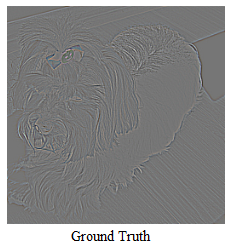## Details of the Example

Our training objective is to learn a function Φ that parameterizes a given discrete image f in a continuous fashion. The image defines a dataset of pixel coordinates Xᵢ = (xᵢ, yᵢ) and their associated RGB values f(Xᵢ). We will apply just a single constraint C(f, Φ) =Φ — f which translates to the loss L which is just the L₂ loss.We train on just the image but also visualize the gradients and the Laplacians. We can straight away see that SIREN is designed not just to map the image, but also its gradients and therefore its Laplacians too.

### Authors Flexing on Us (Solving the Poisson’s Equation)

We saw how the proposed representation can accurately represent an image and its derivatives. Now, instead of training on the image, what if we train on its gradients i.e. what if we train the implicit representation network based on a loss function which only contains the gradients of the image? Well, the authors did that too 😃. (NOTE: Here we are training the weights of the function itself, but the loss contains the derivatives. This means that the network never gets to see the actual image).

If you thought that they would stop at that, you were mistaken. They went ahead and trained on just the Laplacians too. This is equivalent to solving the Poisson’s equation:Solving the Poisson’s equation just involves going back from the Laplacian to the function.

The results were surprising.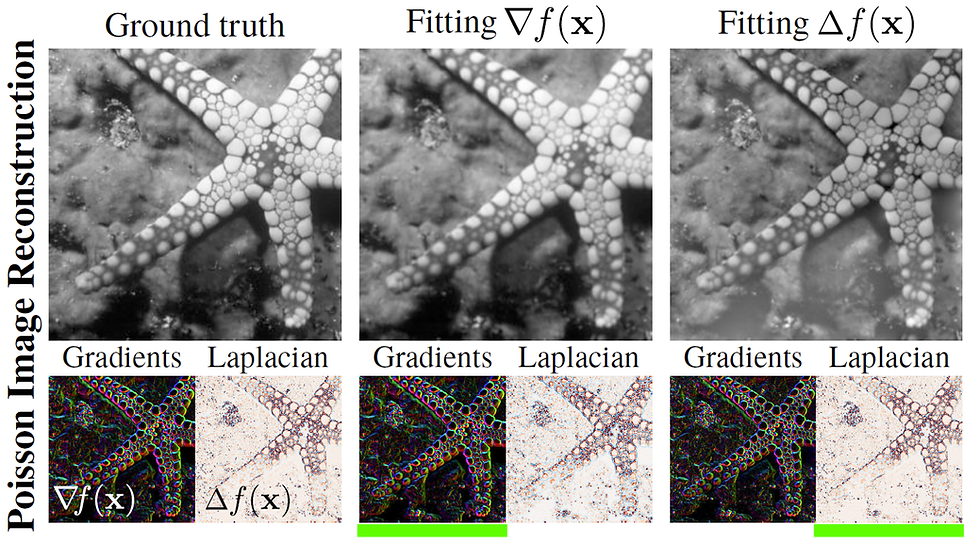Image reconstructed by fitting a SIREN supervised either by its gradients or Laplacians (underlined in green).

Keep in mind that nothing even remotely similar can be done with a ReLU based MLP as ReLU has a constant derivative and no Laplacian.

The derivative of a SIREN is also a SIREN cosine is just a shifted sine. No other commonly used non-linearities such as tanh or ReLU has this property. This becomes useful when we want to match not just the image, but its derivatives too.

DISCLAIMER: At the moment, this only works with B&W images. On integration, we lose bias information and for RGB images with three channels, colour distortions occur. For B&W images, we only get distortions in luminosity.

## More Flexing (Fusion of Images)

Even if you wanted to create a fusion of two monochrome images, the authors got you covered. If we directly combine the two images by taking average pixel values, we will just get a washed-out image.

Now, all the ‘GAN-fans’ must be getting riled up, but even for GANs, we need a training dataset 😇.

The authors took the gradients of the two images, fused them together, and then trained the image on the composite gradients as before.## General Case (a.k.a. Math Time)

As you have seen, SIRENs can fit anything that can be formulated as a set of constraints that relate the input of the function to its output or any of the derivatives of the output.

Thus, we are interested in a class of functions Φ that satisfy equations of the form: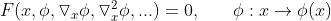For the implicit representation network trained on the image, this would be of the form:For the network trained on just the gradients of the image, the above equation would become:Similarly, for the network trained on just the Laplacians of the image, it is:Therefore, our goal is to learn a neural network that parameterizes Φ to map x to some quantity of interest while satisfying the constraints. We can cast this as a feasibility problem where a function Φ is to be found which satisfies a set of M constraints:In our Terrier example, the constraint was C(f, Φ) =Φ — f. But due to the amazing power of SIRENs, we can impose multiple and much more complex constraints to fit harder datasets.

Each of the M constraints relate the function Φ and/or its derivatives to quantities a(x). A constraint is met when its value becomes zero. This problem can be cast in a loss function that penalizes deviations from each of the constraints on their domain Ωₘ.The indicator function 1 is equal to 1 when x ∈ Ωₘ and 0 when x ∉ Ωₘ.

In practice, the dataset is sampled dynamically at training time, approximating L better as the number of samples grow. We parameterize the functions Φ as fully connected neural networks with parameters θ and solve the resulting optimization problem using gradient descent.

## A disadvantage of SIREN and The Solution

The sine function is periodic. So, while training, if you want to go up the hill and you take a step too large, then you will end up down the hill again. This is a disadvantage. The authors get around this by applying a specific kind of initialization. This is necessary for the effective training of SIRENs.

The initialization is done so as to preserve the distribution of activations through the layer such that the final output at initialization is independent of the number of layers.

Each component of w (the weights) is uniformly distributed such that wᵢ ~ U(-√(6/n), √(6/n)). This ensures that the input to each sine activation is normally distributed with a standard deviation of 1. Also, they initialize the first layer of the sine network with weights so that the function sin(W₀ . W + b) spans multiple periods over [-1,1]. The authors found W₀ = 30 to work well for their applications. More details about how they arrived at this initialization scheme can be found in the paper.

## Other Applications

### Representing Shapes with Signed Distance Functions

A point cloud is a set of data points in 3D space. These points may represent shapes in space. To get an intuition, you can think of the light dots that an Xbox Kinect projects into your room to get the 3D model of its surroundings. Below are some visual representation of point cloud data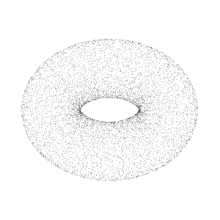An SDF (Signed Distance Function) is a metric which measures the distance of a point x (of the point cloud) from the boundary Ω. It can be used to train an implicit representation network to fit point clouds. The sign of SDF at a point indicates which side of the boundary the point is.

So we are going from point clouds to shapes by training an implicit representation i.e. a neural network that represents the shape by mapping coordinates to signed distance values.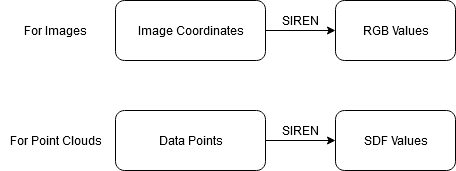As mentioned before, the amazing power of SIRENs enables us to impose multiple constraints. This amounts to solving a particular ‘Eikonal boundary value problem’. Here, the main constraint is that the norm of the spatial gradients |∇ₓ Φ| should be 1 everywhere. The intuition behind this is as follows: the SDF is just the distance to the closest point, so the gradient will point in the opposite direction to that point. Also, if we move one unit away from that point, the SDF will increase by 1. Therefore, the gradient is one.The loss function used is:We will analyze this loss part by part.

I — This part says that the gradient should be 1 as discussed before

II — This part demands two things. Firstly, for points on the surface, the SDF should be zero. Second, the gradient of the SDF and the normal vector at that point should align. This makes sense because we do not want the SDF to increase along the surface (because it is zero at all points on surface).

III — This part penalizes off-surface points for creating SDF values close to zero. Here Ψ(x) = exp(-α . |Φ(x)|), α >> 1.

ReLU MLP and SIREN were trained on these constraints. Here too, SIREN outperforms ReLU based networks.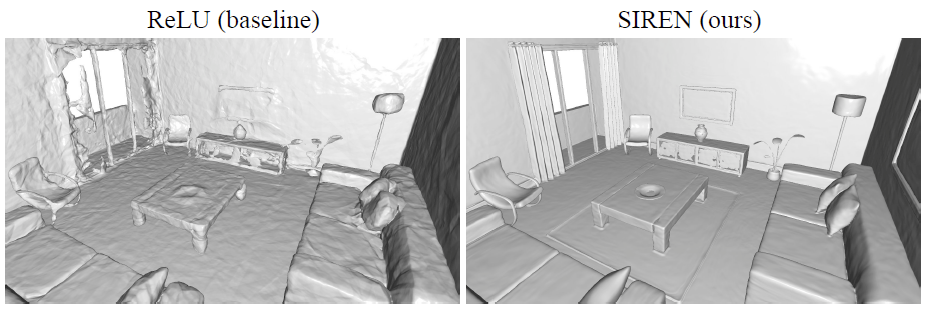Significantly better details and complexity of entire scenes for SIREN

## Conclusion

As you have seen, SIREN allows for an accurate representation of images. In addition, it can also be used for other natural signals such as audio and video in a deep learning framework. There are several exciting avenues for future research on SIRENs, including improvement in the performance of sinusoidal activation networks and finding applications in areas beyond implicit neural representations. I would highly recommend everyone to take a look at the SIREN paper as it is very well written. Also, check out the paper website as it contains many more applications and interesting visuals.

# Content Sources:

1. The SIREN paper — https://arxiv.org/abs/2006.09661

2. The website for the paper — https://vsitzmann.github.io/siren/

3. Official Video Documentation — https://youtu.be/Q2fLWGBeaiI

4. Yannic Kilcher’s Youtube Channel — https://youtu.be/Q5g3p9Zwjrk

5. scart97’s github repo on SIREN — https://github.com/scart97/Siren-fastai2